# Frank Solutions Class 9 Maths Chapter 18 Rectilinear Figures

Frank Solutions Class 9 Maths Chapter 18 Rectilinear Figures help students with 100% accurate answers, along with shortcut tips and tricks. Those who find Maths difficult are advised to practise Frank Solutions thoroughly. Practising textbook problems using these solutions improves conceptual knowledge among students, undoubtedly. It also boosts confidence and skills in solving problems quickly. Access the Frank Solutions Class 9 Maths Chapter 18 Rectilinear Figures PDF, both online and offline, from the link provided below.

Chapter 18 Rectilinear Figures has perfect answers provided for the questions in the textbook. A figure, all of whose edge intersections are at right angles, is known as a rectilinear figure. Students can use Frank Solutions on a daily basis, as they provide vital questions that may arise in examinations. They can also check whether their answers are correct or not while revising the textbook problems.

## Frank Solutions for Class 9 Maths Chapter 18 Rectilinear Figures – Download the PDF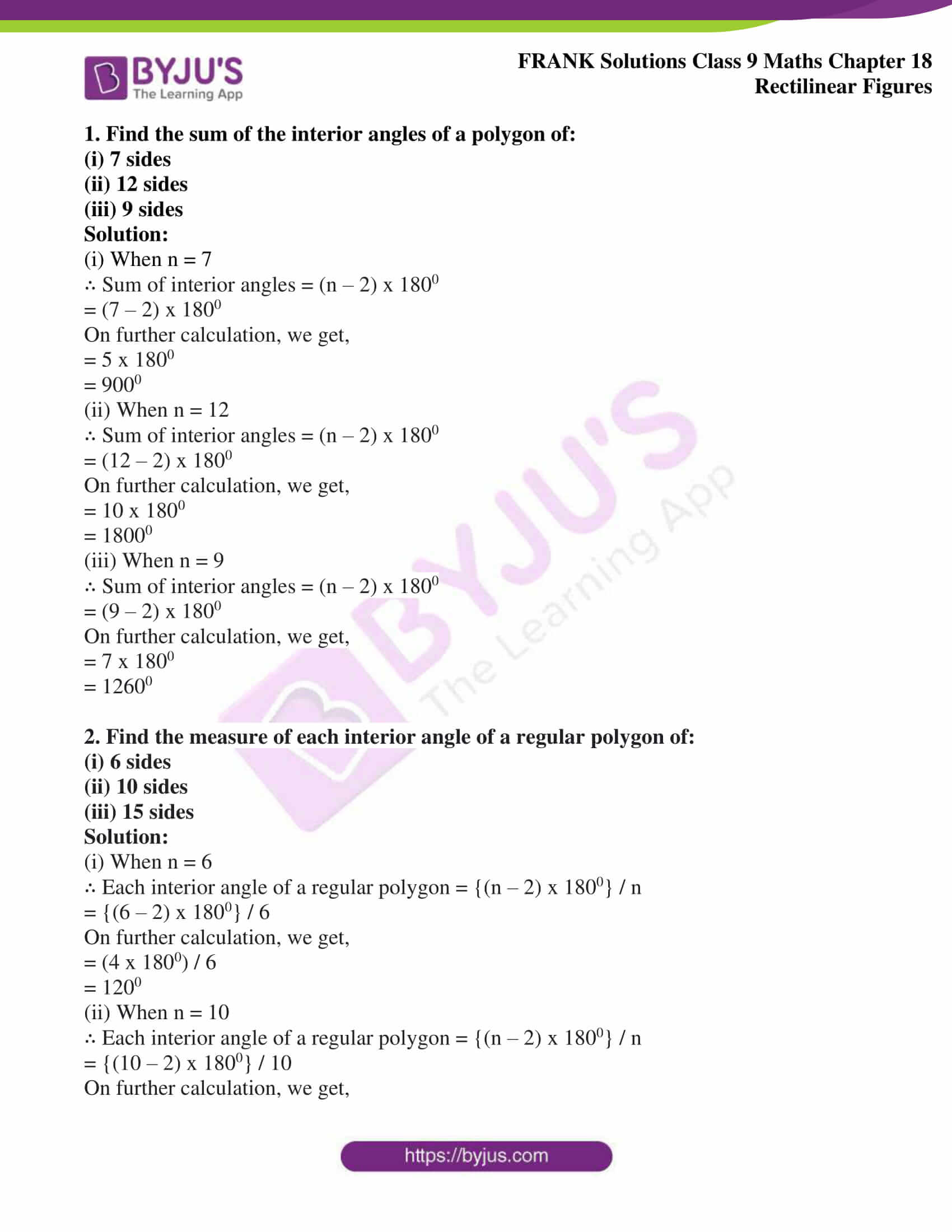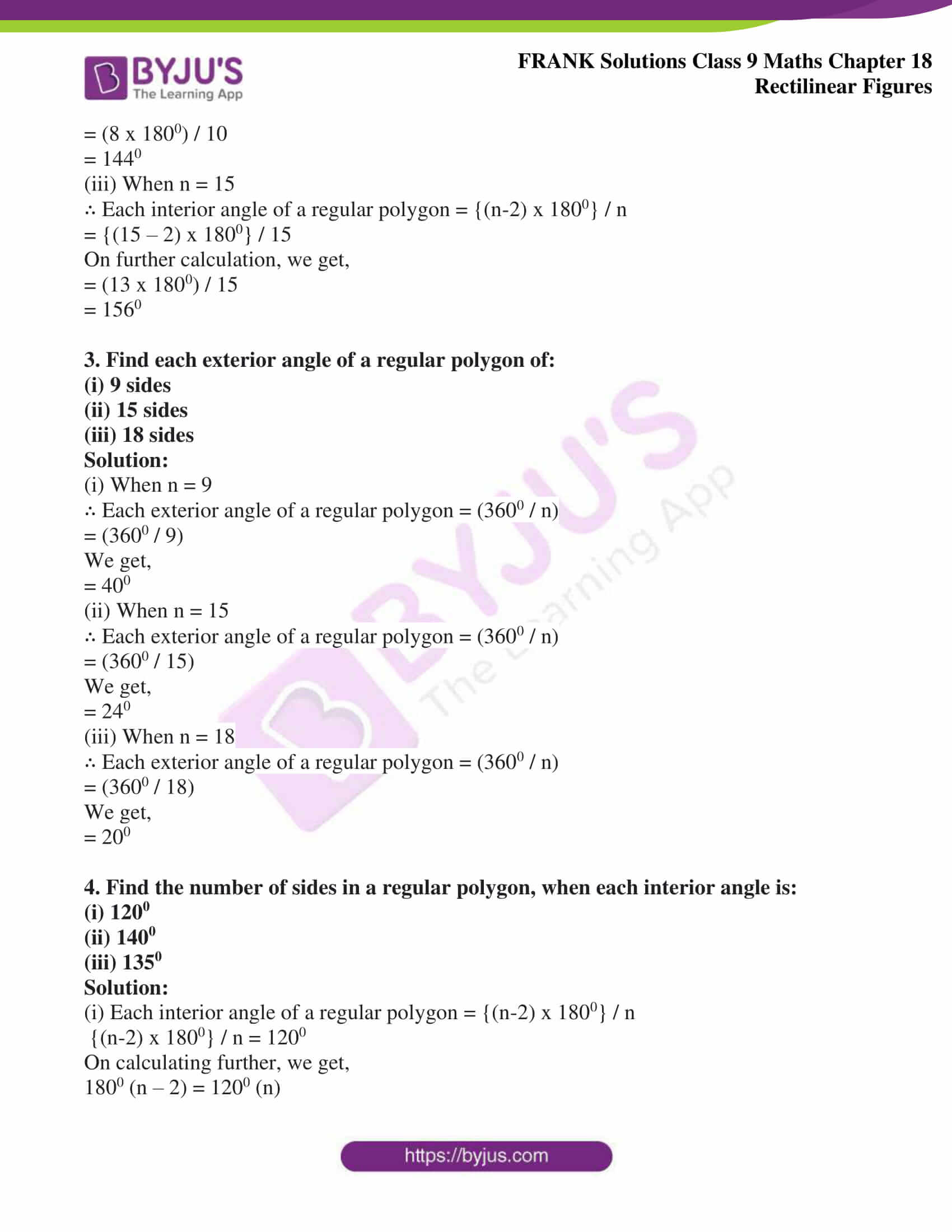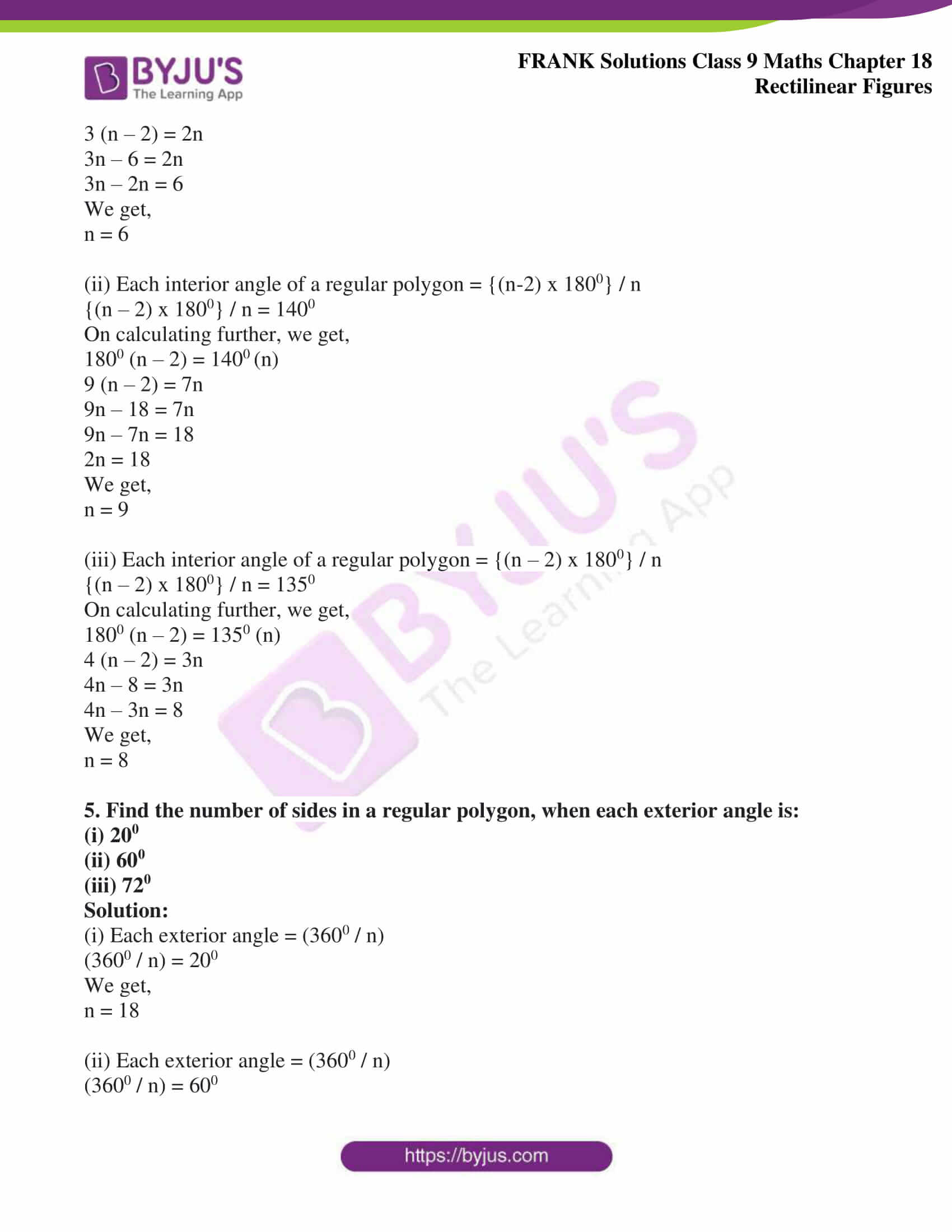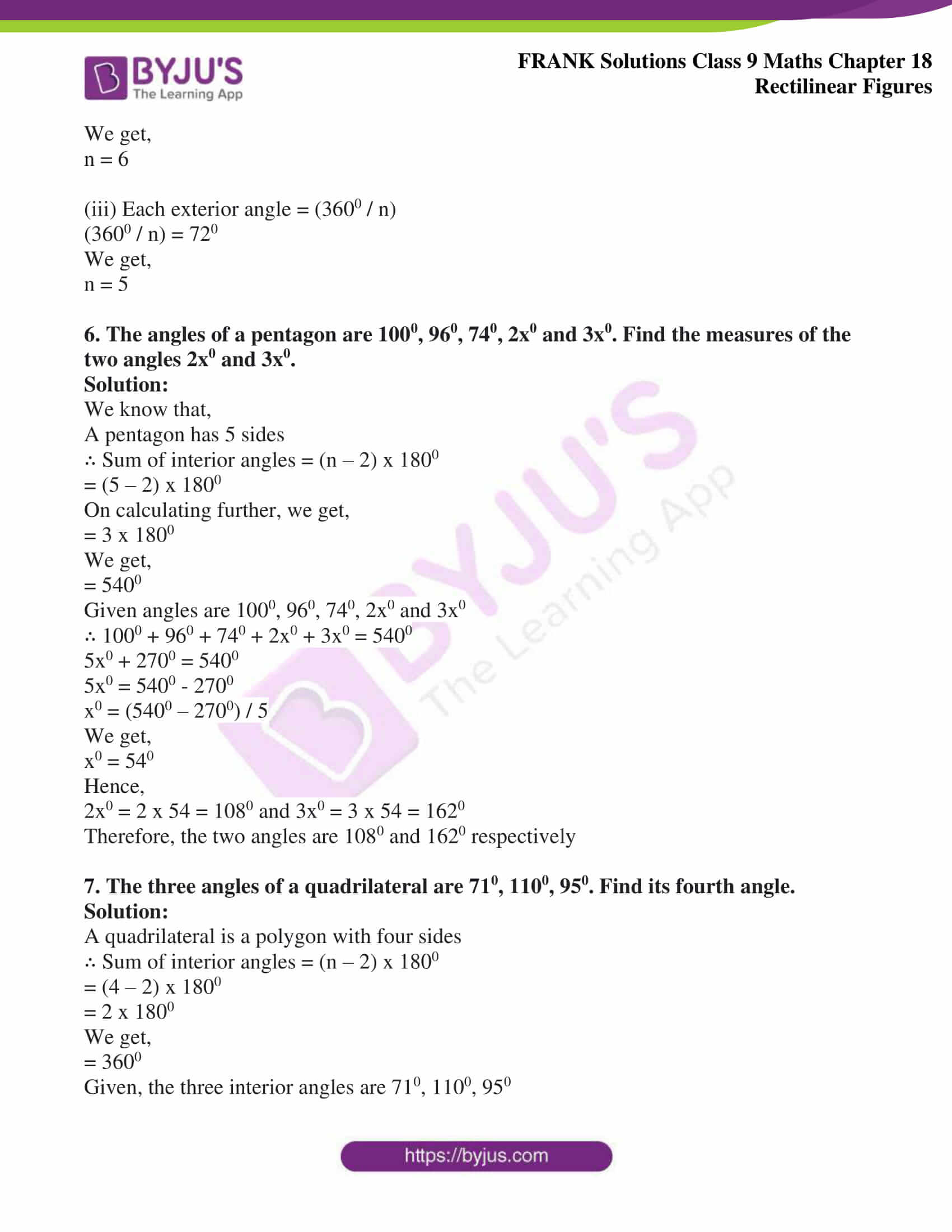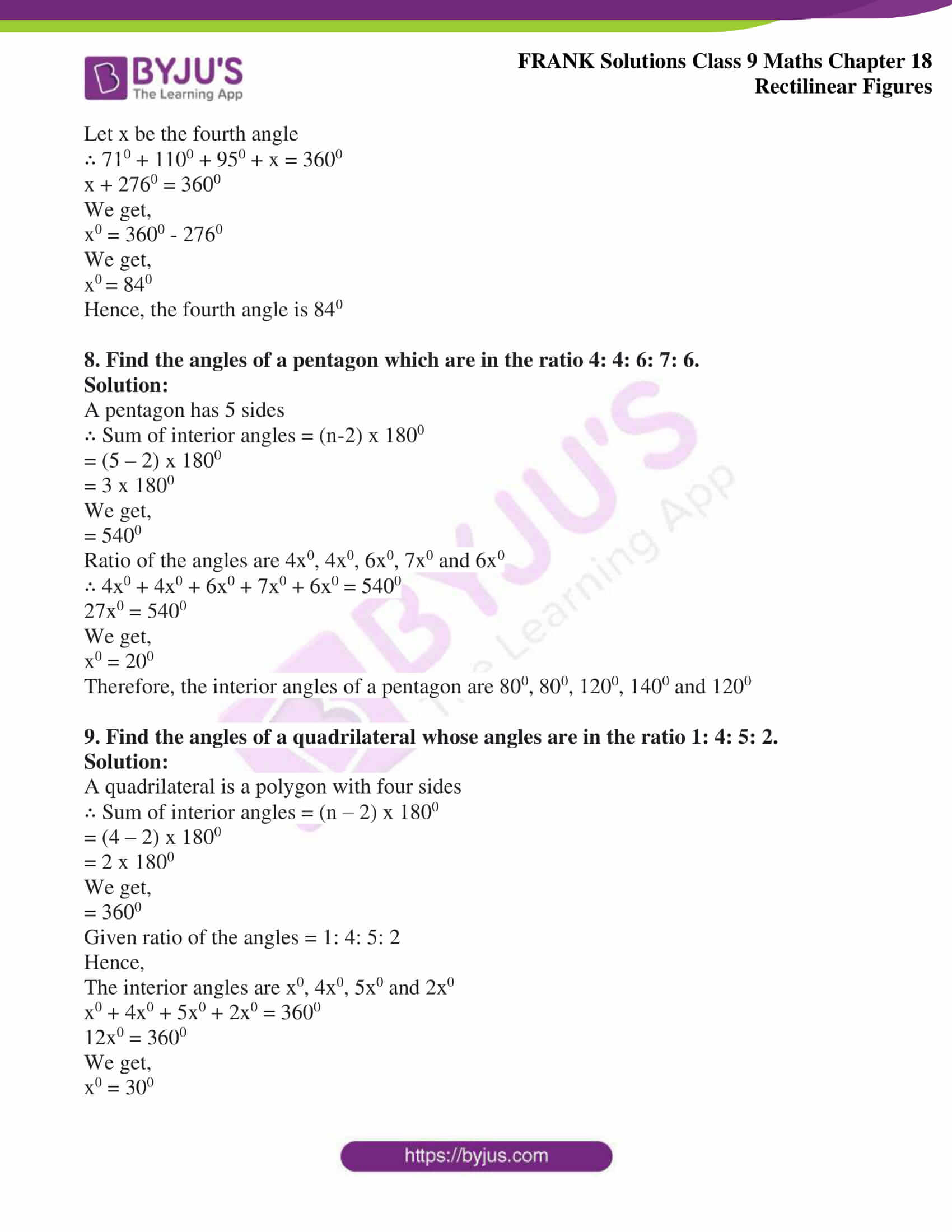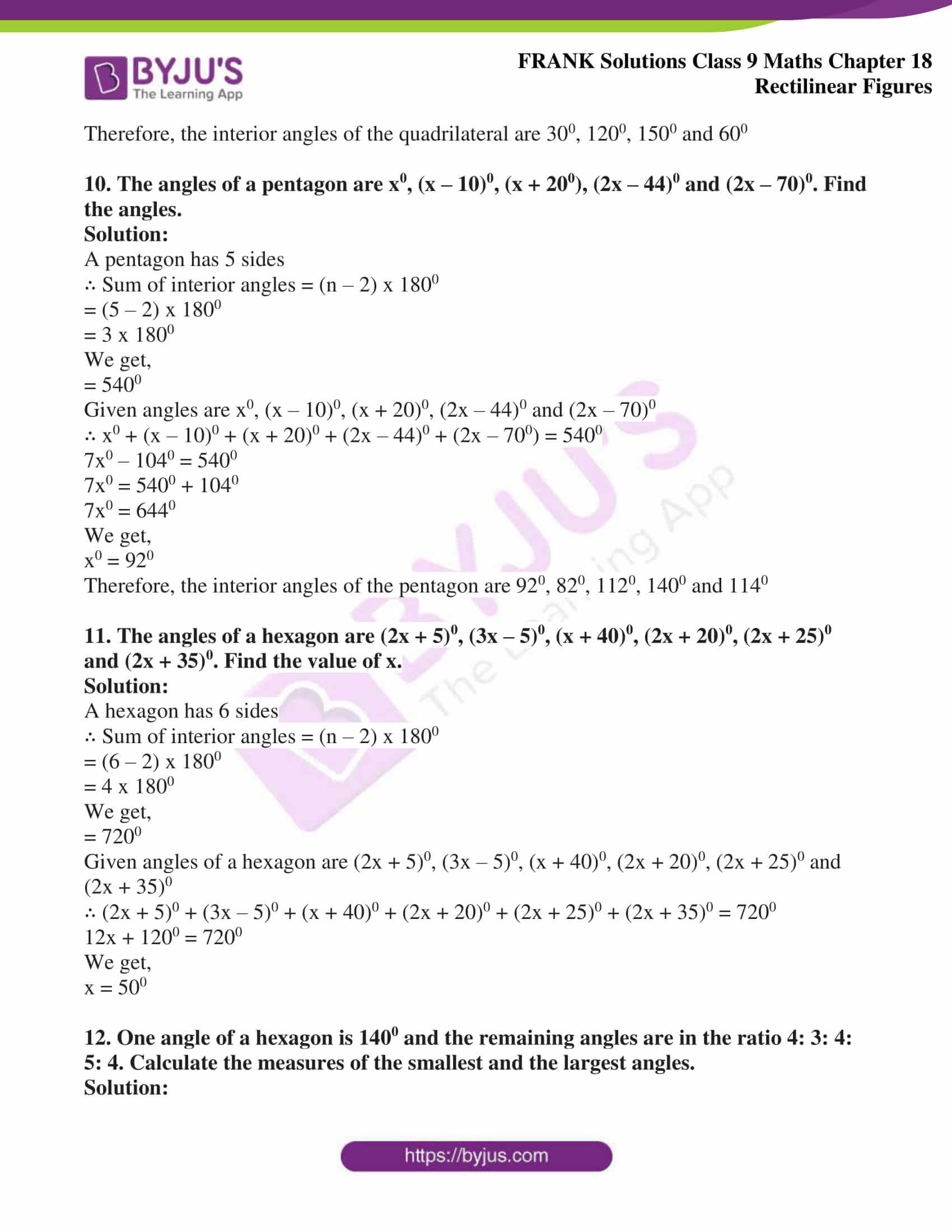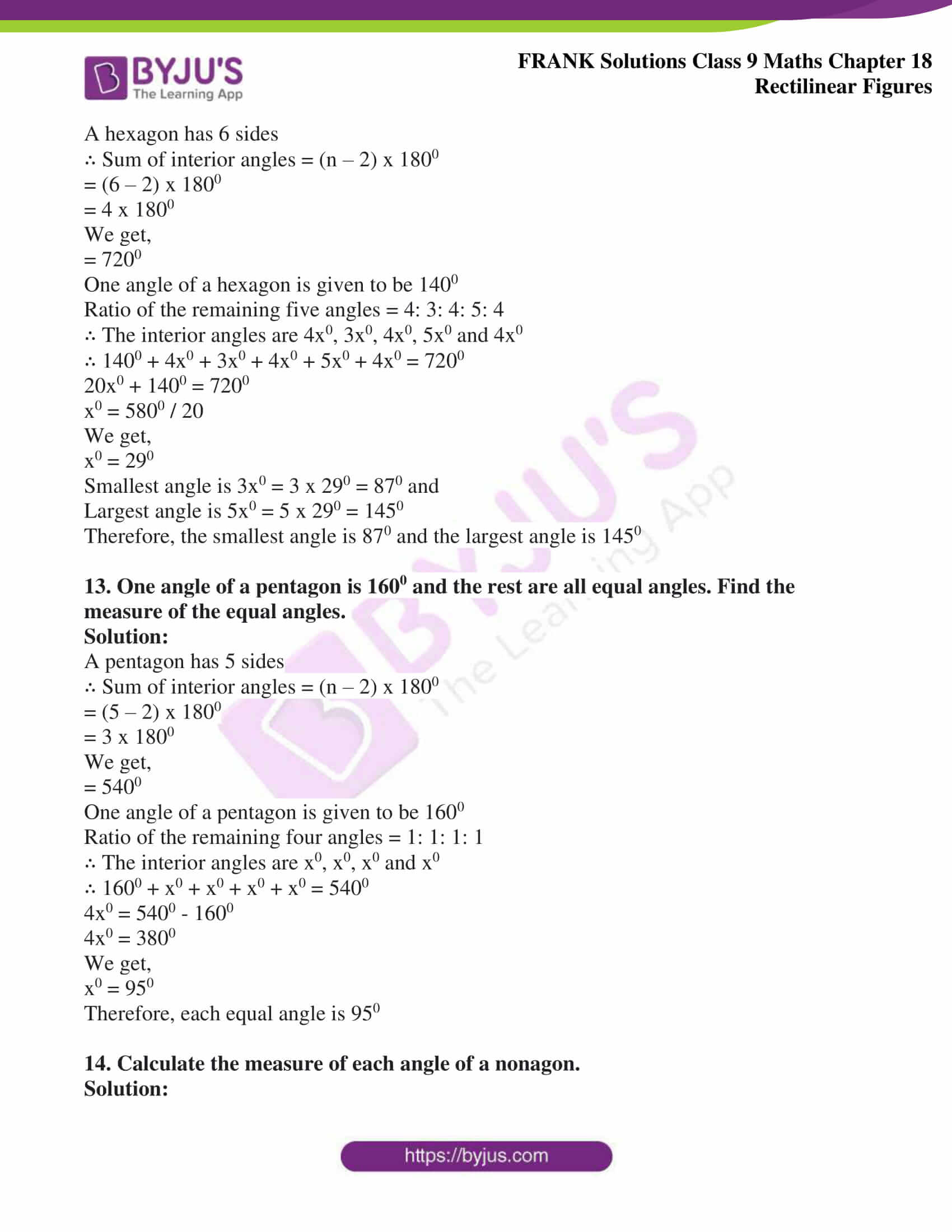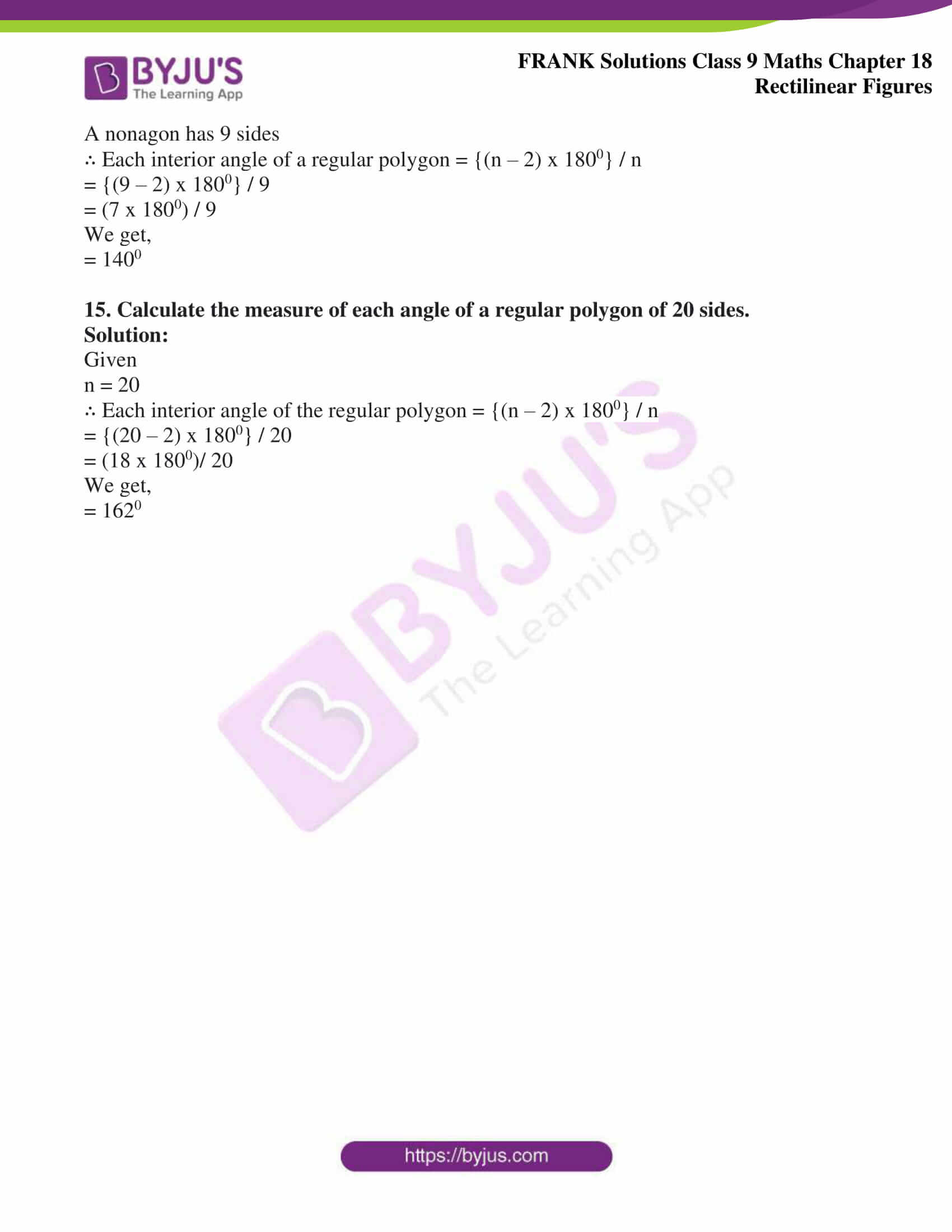## Access Frank Solutions for Class 9 Maths Chapter 18 Rectilinear Figures

1. Find the sum of the interior angles of a polygon of:

(i) 7 sides

(ii) 12 sides

(iii) 9 sides

Solution:

(i) When n = 7

∴ Sum of interior angles = (n – 2) x 1800

= (7 – 2) x 1800

On further calculation, we get,

= 5 x 1800

= 9000

(ii) When n = 12

∴ Sum of interior angles = (n – 2) x 1800

= (12 – 2) x 1800

On further calculation, we get,

= 10 x 1800

= 18000

(iii) When n = 9

∴ Sum of interior angles = (n – 2) x 1800

= (9 – 2) x 1800

On further calculation, we get,

= 7 x 1800

= 12600

2. Find the measure of each interior angle of a regular polygon of:

(i) 6 sides

(ii) 10 sides

(iii) 15 sides

Solution:

(i) When n = 6

∴ Each interior angle of a regular polygon = {(n – 2) x 1800} / n

= {(6 – 2) x 1800} / 6

On further calculation, we get,

= (4 x 1800) / 6

= 1200

(ii) When n = 10

∴ Each interior angle of a regular polygon = {(n – 2) x 1800} / n

= {(10 – 2) x 1800} / 10

On further calculation, we get,

= (8 x 1800) / 10

= 1440

(iii) When n = 15

∴ Each interior angle of a regular polygon = {(n-2) x 1800} / n

= {(15 – 2) x 1800} / 15

On further calculation, we get,

= (13 x 1800) / 15

= 1560

3. Find each exterior angle of a regular polygon of:

(i) 9 sides

(ii) 15 sides

(iii) 18 sides

Solution:

(i) When n = 9

∴ Each exterior angle of a regular polygon = (3600 / n)

= (3600 / 9)

We get,

= 400

(ii) When n = 15

∴ Each exterior angle of a regular polygon = (3600 / n)

= (3600 / 15)

We get,

= 240

(iii) When n = 18

∴ Each exterior angle of a regular polygon = (3600 / n)

= (3600 / 18)

We get,

= 200

4. Find the number of sides in a regular polygon, when each interior angle is:

(i) 1200

(ii) 1400

(iii) 1350

Solution:

(i) Each interior angle of a regular polygon = {(n-2) x 1800} / n

{(n-2) x 1800} / n = 1200

On calculating further, we get,

1800 (n – 2) = 1200 (n)

3 (n – 2) = 2n

3n – 6 = 2n

3n – 2n = 6

We get,

n = 6

(ii) Each interior angle of a regular polygon = {(n-2) x 1800} / n

{(n – 2) x 1800} / n = 1400

On calculating further, we get,

1800 (n – 2) = 1400 (n)

9 (n – 2) = 7n

9n – 18 = 7n

9n – 7n = 18

2n = 18

We get,

n = 9

(iii) Each interior angle of a regular polygon = {(n – 2) x 1800} / n

{(n – 2) x 1800} / n = 1350

On calculating further, we get,

1800 (n – 2) = 1350 (n)

4 (n – 2) = 3n

4n – 8 = 3n

4n – 3n = 8

We get,

n = 8

5. Find the number of sides in a regular polygon, when each exterior angle is:

(i) 200

(ii) 600

(iii) 720

Solution:

(i) Each exterior angle = (3600 / n)

(3600 / n) = 200

We get,

n = 18

(ii) Each exterior angle = (3600 / n)

(3600 / n) = 600

We get,

n = 6

(iii) Each exterior angle = (3600 / n)

(3600 / n) = 720

We get,

n = 5

6. The angles of a pentagon are 1000, 960, 740, 2x0 and 3x0. Find the measures of the two angles 2x0 and 3x0.

Solution:

We know that,

A pentagon has 5 sides

∴ Sum of interior angles = (n – 2) x 1800

= (5 – 2) x 1800

On calculating further, we get,

= 3 x 1800

We get,

= 5400

Given angles are 1000, 960, 740, 2x0 and 3x0

∴ 1000 + 960 + 740 + 2x0 + 3x0 = 5400

5x0 + 2700 = 5400

5x0 = 5400 – 2700

x0 = (5400 – 2700) / 5

We get,

x0 = 540

Hence,

2x0 = 2 x 54 = 1080 and 3x0 = 3 x 54 = 1620

Therefore, the two angles are 1080 and 1620 respectively

7. The three angles of a quadrilateral are 710, 1100, 950. Find its fourth angle.

Solution:

A quadrilateral is a polygon with four sides

∴ Sum of interior angles = (n – 2) x 1800

= (4 – 2) x 1800

= 2 x 1800

We get,

= 3600

Given, the three interior angles are 710, 1100, 950

Let x be the fourth angle

∴ 710 + 1100 + 950 + x = 3600

x + 2760 = 3600

We get,

x0 = 3600 – 2760

We get,

x0 = 840

Hence, the fourth angle is 840

8. Find the angles of a pentagon which are in the ratio 4: 4: 6: 7: 6.

Solution:

A pentagon has 5 sides

∴ Sum of interior angles = (n-2) x 1800

= (5 – 2) x 1800

= 3 x 1800

We get,

= 5400

Ratio of the angles are 4x0, 4x0, 6x0, 7x0 and 6x0

∴ 4x0 + 4x0 + 6x0 + 7x0 + 6x0 = 5400

27x0 = 5400

We get,

x0 = 200

Therefore, the interior angles of a pentagon are 800, 800, 1200, 1400 and 1200

9. Find the angles of a quadrilateral whose angles are in the ratio 1: 4: 5: 2.

Solution:

A quadrilateral is a polygon with four sides

∴ Sum of interior angles = (n – 2) x 1800

= (4 – 2) x 1800

= 2 x 1800

We get,

= 3600

Given ratio of the angles = 1: 4: 5: 2

Hence,

The interior angles are x0, 4x0, 5x0 and 2x0

x0 + 4x0 + 5x0 + 2x0 = 3600

12x0 = 3600

We get,

x0 = 300

Therefore, the interior angles of the quadrilateral are 300, 1200, 1500 and 600

10. The angles of a pentagon are x0, (x – 10)0, (x + 200), (2x – 44)0 and (2x – 70)0. Find the angles.

Solution:

A pentagon has 5 sides

∴ Sum of interior angles = (n – 2) x 1800

= (5 – 2) x 1800

= 3 x 1800

We get,

= 5400

Given angles are x0, (x – 10)0, (x + 20)0, (2x – 44)0 and (2x – 70)0

∴ x0 + (x – 10)0 + (x + 20)0 + (2x – 44)0 + (2x – 700) = 5400

7x0 – 1040 = 5400

7x0 = 5400 + 1040

7x0 = 6440

We get,

x0 = 920

Therefore, the interior angles of the pentagon are 920, 820, 1120, 1400 and 1140

11. The angles of a hexagon are (2x + 5)0, (3x – 5)0, (x + 40)0, (2x + 20)0, (2x + 25)0 and (2x + 35)0. Find the value of x.

Solution:

A hexagon has 6 sides

∴ Sum of interior angles = (n – 2) x 1800

= (6 – 2) x 1800

= 4 x 1800

We get,

= 7200

Given angles of a hexagon are (2x + 5)0, (3x – 5)0, (x + 40)0, (2x + 20)0, (2x + 25)0 and (2x + 35)0

∴ (2x + 5)0 + (3x – 5)0 + (x + 40)0 + (2x + 20)0 + (2x + 25)0 + (2x + 35)0 = 7200

12x + 1200 = 7200

We get,

x = 500

12. One angle of a hexagon is 1400 and the remaining angles are in the ratio 4: 3: 4: 5: 4. Calculate the measures of the smallest and the largest angles.

Solution:

A hexagon has 6 sides

∴ Sum of interior angles = (n – 2) x 1800

= (6 – 2) x 1800

= 4 x 1800

We get,

= 7200

One angle of a hexagon is given to be 1400

Ratio of the remaining five angles = 4: 3: 4: 5: 4

∴ The interior angles are 4x0, 3x0, 4x0, 5x0 and 4x0

∴ 1400 + 4x0 + 3x0 + 4x0 + 5x0 + 4x0 = 7200

20x0 + 1400 = 7200

x0 = 5800 / 20

We get,

x0 = 290

Smallest angle is 3x0 = 3 x 290 = 870 and

Largest angle is 5x0 = 5 x 290 = 1450

Therefore, the smallest angle is 870 and the largest angle is 1450

13. One angle of a pentagon is 1600 and the rest are all equal angles. Find the measure of the equal angles.

Solution:

A pentagon has 5 sides

∴ Sum of interior angles = (n – 2) x 1800

= (5 – 2) x 1800

= 3 x 1800

We get,

= 5400

One angle of a pentagon is given to be 1600

Ratio of the remaining four angles = 1: 1: 1: 1

∴ The interior angles are x0, x0, x0 and x0

∴ 1600 + x0 + x0 + x0 + x0 = 5400

4x0 = 5400 – 1600

4x0 = 3800

We get,

x0 = 950

Therefore, each equal angle is 950

14. Calculate the measure of each angle of a nonagon.

Solution:

A nonagon has 9 sides

∴ Each interior angle of a regular polygon = {(n – 2) x 1800} / n

= {(9 – 2) x 1800} / 9

= (7 x 1800) / 9

We get,

= 1400

15. Calculate the measure of each angle of a regular polygon of 20 sides.

Solution:

Given

n = 20

∴ Each interior angle of the regular polygon = {(n – 2) x 1800} / n

= {(20 – 2) x 1800} / 20

= (18 x 1800)/ 20

We get,

= 1620